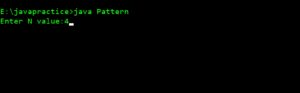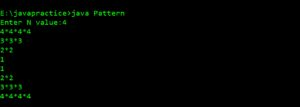# Print Number Star Half Diamond Pattern Type 3

## PRINT PATTERN  4*4*4*4 3*3*3 2*2 1

For any input number N Print the following code – For below code N=4

```4*4*4*4
3*3*3
2*2
1
1
2*2
3*3*3
4*4*4*4```

### PREREQUISITE:

Basic knowledge in Java programming, usage of loops.

### ALGORITHM:

1. Take input from user i.e number of lines required (N value).
2. Take two loops one for each line (say ‘i’) and other for each digit in a particular line (say ‘j’). i starts from 1 and j starts from 1.
3. The program is divided into two sections for decrement part (i.e first 4 lines) and increment part (last 4 lines). Two separate i and j loops are written for each part. In first part i is from n to 1 and in the second part i is from 1 to n.
4. Here ‘i’ loop is used to access each line from 1 to n and ‘j’ loop is used to print values in each line. j loop is executed until it reaches i value.
5. Print ‘i’ value until the j loop reaches i value.
6. Print the final value ‘i’ of each line and go to next line.

### CODE IN JAVA:

[code language=”java”]
import java.lang.*;
import java.io.*;
class Pattern
{
public static void main(String[] args)throws IOException
{
int n,i,j;
System.out.print("Enter N value:");
for(i=n;i>=1;i–)
{
for(j=1;j<i;j++)
{
System.out.print(i+"*");
}
System.out.println(i);
}
for(i=1;i<=n;i++)
{
for(j=1;j<i;j++)
{
System.out.print(i+"*");
}
System.out.println(i);
}
}
}
[/code]

### TAKING INPUT:### DISPLAYING THE OUTPUT: